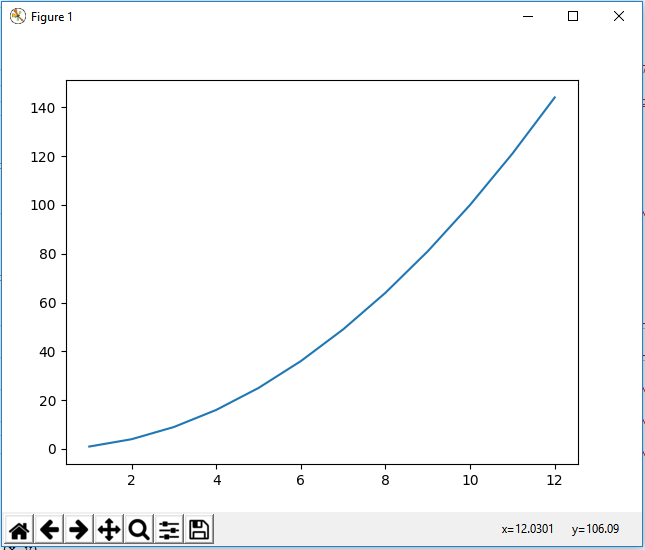﻿ How to Create a Graph Plot in Matplotlib with Python ﻿# How to Create a Graph Plot in Matplotlib with PythonIn this article, we show how to create a graph plot in matplotlib with Python.

So with matplotlib, the heart of it is to create a figure.

On this figure, you can populate it with all different types of data, including axes, a graph plot, a geometric shape, etc.

How can we add a graph plot to this figure in matplotlib?

We can do this in matplotlib using the plot() function.

So the first thing we have to do is import matplotlib. We do this with the line, import matplotlib.pyplot as plt

We then create a variable fig, and set it equal to, plt.figure()

This creates a figure object, which of course is initially empty, because we haven't populated it with anything.

So we have a figure object stored in the variable, fig, that is empty.

This add_axes() function takes in a list of values. The values from the left to right are the left, bottom, width, and height.

They are numbers that vary from 0 to 1 and represent the percentage of the blank figure that you want to go and take.

So for this figure in our code, we have the line, axes= fig.add_axes([0.1,0.1,0.8,0.8]) This adds axes to the figure.

We then define the points that we are going to plot.

The x-axis is composed of the x list. This is a list from 1 to 12.

The y-axis is composed of the y list. This is a list from 12 to 144 (the square of each of the x values).

We then plot the points with the plot() function. We use axes.plot(x,y) to plot the x and y values in their lists.

To show this figure object, we use the line, fig.show()

This works if you're using a python IDE other than jupyter notebooks. If you are using jupyter notebooks, then you would not use, plt.show(). Instead you would specify in the code right after importing matplotlib, %matplotlib inline

This line allows the figure of a graph to be shown with jupyter notebooks.

After running the following code above, we get the following figure shown in the image below.So now you see a figure object with the numbers from 1 to 12 shown on the x-axis and from 12 to 144 shown on the y-axis.

And this is how to create a graph plot in matplotlib with Python.

Related Resources

How to Randomly Select From or Shuffle a List in Python